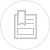# anodic reactions and the tendency to corrosion

For a series of given conditions, the potential is represented by the Nernst equation (see oxidation-reduction and basic priniciples - definitions). The Nernst equation applied to anodic corrosion at 25°C is expressed thus :

where :

Eao = the anodic reaction’s normal potential ;

M = activity of the metal ion in the solution.

Table 15 in chapter fundamental physical-chemical engineering processes applicable to water treatment defines the classification applicable to the normal potential of a range of metals according to their normal hydrogen potential. Metals having a positive normal potential are designated "noble" and are less prone to corrosion. Metals having a lower normal potential are less noble. Metals having a negative normal potential are thermodynamically unstable in the presence of pure water with a pH of 7.0 and a temperature of 25°C. We can quote iron, that has a normal potential of – 0.44 volt and, therefore, is not a noble metal, and copper that has a potential of + 0.34 volt and is noble.